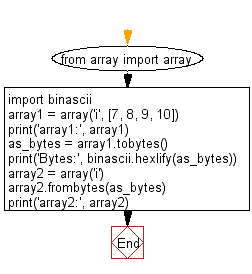﻿ Python: Read a string and interpreting the string as an array of machine values - w3resource

# Python: Read a string and interpreting the string as an array of machine values

## Python: Array Exercise-22 with Solution

Write a Python program that reads a string and interprets it as an array of machine values.

Sample Solution:

Python Code:

``````from array import array
import binascii
array1 = array('i', [7, 8, 9, 10])
print('array1:', array1)
as_bytes = array1.tobytes()
print('Bytes:', binascii.hexlify(as_bytes))
array2 = array('i')
array2.frombytes(as_bytes)
print('array2:', array2)
```
```

Sample Output:

```array1: array('i', [7, 8, 9, 10])
Bytes: b'0700000008000000090000000a000000'
array2: array('i', [7, 8, 9, 10])
```

Flowchart:Python Code Editor: